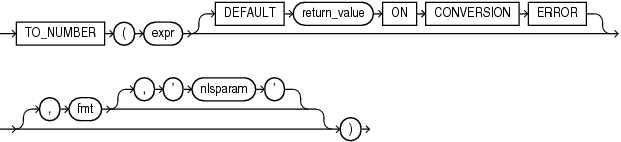## TO_NUMBER

SyntaxDescription of the illustration to_number.eps

Purpose

`TO_NUMBER` converts `expr` to a value of `NUMBER` data type.

`expr` can be any expression that evaluates to a character string of type `CHAR`, `VARCHAR2`, `NCHAR`, or `NVARCHAR2`, a numeric value of type `NUMBER`, `BINARY_FLOAT`, or `BINARY_DOUBLE`, or null. If `expr` is `NUMBER`, then the function returns `expr`. If `expr` evaluates to null, then the function returns null. Otherwise, the function converts `expr` to a `NUMBER` value.

• If you specify an `expr` of `CHAR`, `VARCHAR2`, `NCHAR`, or `NVARCHAR2` data type, then you can optionally specify the format model `fmt`.

• If you specify an `expr` of `BINARY_FLOAT` or `BINARY_DOUBLE` data type, then you cannot specify a format model because a float can be interpreted only by its internal representation.

Refer to for information on number formats.

The `'nlsparam'` argument in this function has the same purpose as it does in the `TO_CHAR` function for number conversions. Refer to TO_CHAR (number) for more information.

This function does not support `CLOB` data directly. However, `CLOB`s can be passed in as arguments through implicit data conversion.

Examples

The following examples convert character string data into a number:

```UPDATE employees SET salary = salary +
TO_NUMBER('100.00', '9G999D99')
WHERE last_name = 'Perkins';
```
```SELECT TO_NUMBER('-AusDollars100','L9G999D99',
' NLS_NUMERIC_CHARACTERS = '',.''
NLS_CURRENCY            = ''AusDollars''
') "Amount"
FROM DUAL;

Amount
----------
-100```

The following example returns the default value of `0` because the specified expression cannot be converted to a `NUMBER` value:

```SELECT TO_NUMBER('2,00' DEFAULT 0 ON CONVERSION ERROR) "Value"
FROM DUAL;

Value
--------
0
```